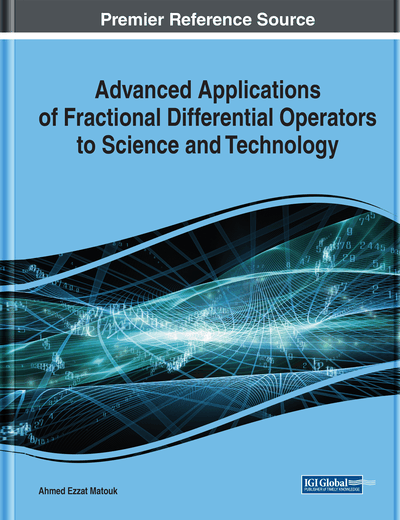# Properties of Spatial-Rotation Transformations of Fractional Derivatives

Ehab Malkawi (Physics Department, United Arab Emirates University, UAE)
DOI: 10.4018/978-1-7998-3122-8.ch009
Available
\$29.50
No Current Special Offers

## Abstract

The transformation properties of the fractional derivatives under spatial rotation in two-dimensional space and for both the Riemann-Liouville and Caputo definitions are investigated and derived in their general form. In particular, the transformation properties of the fractional derivatives acting on scalar fields are studied and discussed. The study of the transformation properties of fractional derivatives is an essential step for the formulation of fractional calculus in multi-dimensional space. The inclusion of fractional calculus in the Lagrangian and Hamiltonian dynamical formulation relies on such transformation. Specific examples on the transformation of the fractional derivatives of scalar fields are discussed.
Chapter Preview
Top

## Introduction

Fractional calculus deals with differentiation and integration to arbitrary real or complex orders. The idea could be traced to the time of the integer-order calculus. For extensive mathematical discussion of fractional calculus, the reader can consult (Samko et al., 1993; Miller & Ross, 1993; Oldham & Spanier, 1974; Nishimoto, 1989; Podlubny, 1999) and references therein. The techniques of fractional calculus have been applied to wide range of fields, such as physics, engineering, chemistry, biology, economics, control theory, signal image processing, groundwater problems, and many others.

Physics applications of fractional calculus span a wide range of topics and problems. For a review of selected physics applications, see (Herrman, 2011; Hilfer, 2000; Carpinteri & Mainardi, 1997; West et al., 2003; Tarasov, 2013; Zaslavsky, 2005) and references therein. Generalizing fractional calculus to several variables, multidimensional space, and generalization of fractional vector calculus has been reported (Hanyga, 2002; Adda, 2001; Engheta, 1998; Naqvi & Abbas, 2004; Meerschaert et al., 2006; Cottrill-Shepard & Naber, 2001; Tarasov, 2005; Yong et al., 2003). In addition, progress has been reported on generalization of Lagrangian and Hamiltonian systems (Riewe, 1996; Herzalla et al., 2011; Golmankhaneh et al., 2012; Muslih & Baleanu, 2005; Klimek, 2002; Agrawal, 2002).

Despite the significant progress in applying fractional calculus to a wide range of physical problems, there is still a lack of satisfactory geometric and physical interpretation, in comparison with the simple interpretations of their integer-order counterparts (see (Podlubny, 2002) and references therein). Effort has been devoted to relate fractional calculus and fractal geometry (Calcgni, 2012; Moshrefi-Torbati & Hammond, 1998; Nigmatullin, 1992; Rutman, 1995; Machado, 2003). A different approach to geometric interpretation of fractional calculus is based on the idea of the contact of α-th order (Adda, 1997). However, a satisfactory interpretation is still missing (Podlubny, 2002).

A study of the symmetry of physical systems described by fractional calculus and investigating the transformation properties of fractional derivative operators under specific groups can shed light on the nature of such operators. For example, studying the transformation of the fractional operators under point transformation (or change of variables) can be found in (Buckwar & Luchko, 1998; Gazizov et al., 2009; Gazizov et al., 2011), even though the class of variables substitution preserving the form of the fractional derivative is found to be very narrow. Generalizing the definition of fractional derivative of a function with respect to another function can be used to study general transformation of point transformation (Gazizov et al., 2012).

## Complete Chapter List

Search this Book:
Reset